# Dispersion method

(diff) ← Older revision | Latest revision (diff) | Newer revision → (diff)

in number theory

A method for solving certain binary equations (binary additive problems) of the form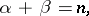(1)

whereandbelong to sequences of natural numbers which are sufficiently dense and sufficiently well distributed in the sequence of natural numbers.

The method, which was developed by Yu.V. Linnik in 1958–1961 and is therefore also known as Linnik's dispersion method, combines elementary concepts of probability theory (in particular, the concept of dispersion and Chebyshev-type inequalities) with the analytic and algebraic ideas of I.M. Vinogradov and A. Weil. It may be described as follows (see also Additive number theory).

Equation (1) may be reduced to equations of the form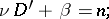(2)

whereandrun, independently of each other, through certain values from a rectangular domain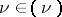,, where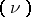and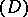are certain intervals; moreover, the numbersare prime, while various additional conditions may be imposed on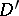. Letbe the number of solutions of this equation.

Now consider the equationfor an arbitrary, and let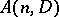be the number of its solutions found by some heuristic means. The (hypothetical) number of expected solutions of equation (2) is then written in the form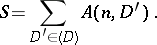An estimate of the difference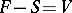has the form(3)

An application of the Cauchy inequality yields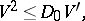(4)

where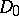is the length of the intervaland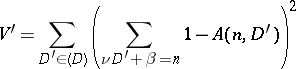(5)

is the dispersion of the number of solutions of equation (2).

If the summation in (5) is extended over all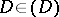, all additional conditions imposed onin (2) are dropped. At the same time the value of the dispersion can only increase; accordingly,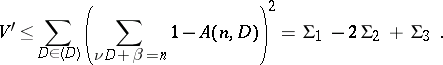The sums,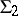and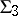can be computed asymptotically in certain cases. The computation of, which is the basic sum in the dispersion method, is the most difficult. The asymptotic computation of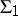is effected using the Vinogradov method by computing, for certain functions, the number of fractional parts comprised in the given segment, and also by using the most recent estimates for trigonometric sums obtained by methods of algebraic geometry. Asymptotics for the sumsandare found by elementary summations. If, as a result, the dispersion is found to be not too large, (3) and (4) yield an asymptotic expansion for the number of solutions of equation (2).

Combining the numbers of solutions of all equations of the type (2) yields an asymptotic formula for the number of solutions of equation (1).

The above scheme of the dispersion method may be used also to solve equations of the typewhereis a given non-zero integer.

Linnik, et al. (see ) employed this method to solve a number of classical additive problems which, prior to the genesis of the dispersion method, could only be solved on the strength of heuristic or hypothetical conceptions. Such problems include the additive divisor problem (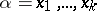,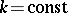,); the Titchmarsh problem on divisors (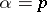is a prime,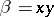); and the Hardy–Littlewood problem (is a prime,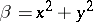).

The dispersion method also yielded solutions of certain analogues and generalizations of these problems; this includes, in particular, an asymptotic expansion for the number of solutions of the general Hardy–Littlewood equation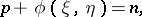whereis a prime number and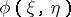is a given primitive positive-definite quadratic form. The existence of an infinite set of prime numbers of the typewhere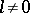is an arbitrary fixed integer, was proved.

The domain of application of the dispersion method intersects with that of Linnik's large sieve method.

How to Cite This Entry:
Dispersion method. Encyclopedia of Mathematics. URL: http://encyclopediaofmath.org/index.php?title=Dispersion_method&oldid=15408
This article was adapted from an original article by B.M. Bredikhin (originator), which appeared in Encyclopedia of Mathematics - ISBN 1402006098. See original article